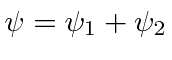## Local Phase Symmetry in Quantum Mechanics and the Gauge Symmetry

There is a symmetry in physics which we might call the Local Phase Symmetry in quantum mechanics. In this symmetry we change the phase of the (electron) wavefunction by a different amount everywhere in spacetime. To compensate for this change, we need to also make a gauge transformation of the electromagnetic potentials. They all must go together like this.The local phase symmetry requires that Electromagnetism exist and have a gauge symmetry so that we can keep the Schrödinger Equation invariant under this phase transformation.

We exploit the gauge symmetry in EM to show that, in field free regions, the functioncan be simply equal to a line integral of the vector potential (if we pick the right gauge).We use this to show that the magnetic flux enclosed by a superconductor is quantized.

We also show that magnetic fields can be used to change interference effects in quantum mechanics. The Aharanov Böhm Effect brings us back to the two slit diffraction experiment but adds magnetic fields.The electron beams travel through two slits in field free regions but we have the ability to vary a magnetic field enclosed by the path of the electrons. At the screen, the amplitudes from the two slits interfere. Let's start withandeverywhere. When we change thefield, the wavefunctions must change.The relative phase from the two slits depends on the flux between the slits. By varying thefield, we will shift the diffraction pattern even thoughalong the whole path of the electrons.

Jim Branson 2013-04-22